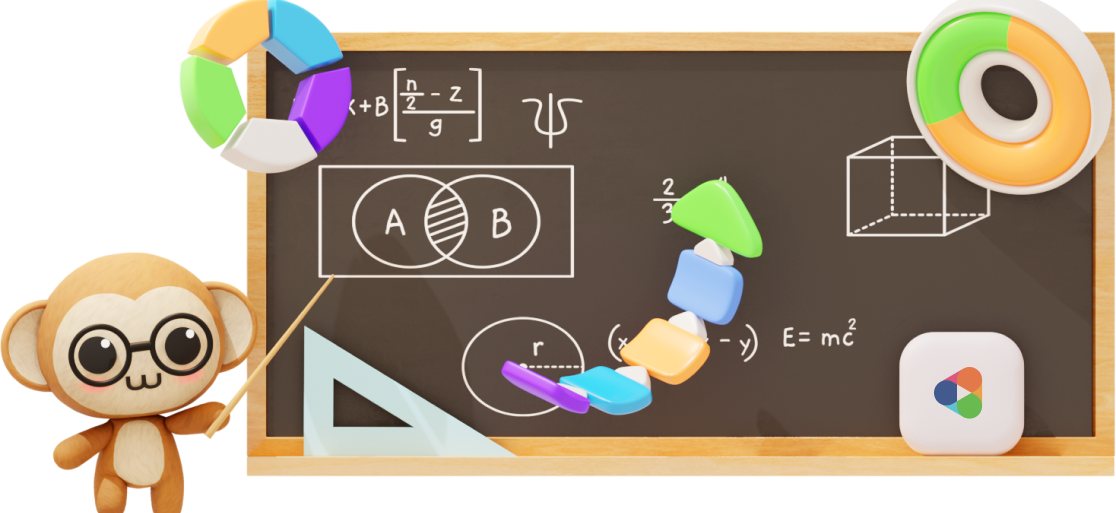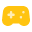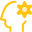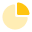# Primary 5 Syllabus

Aligned with the latest 2021 Singapore MOE Math syllabus and endorsed by Education Alliance of Finland.
Hundreds of hours of learning content for all topics covered in P5 to get your child build a strong Math foundation!## Overview

P5 is a difficult year for many students.

Having just made it through a trying year of transitioning into upper primary math in P4, they now face the onslaught of PSLE preparations.

Not to worry, because we have plenty of fun and effective learning material to help them build their skills and confidence, and put your mind at ease.

Find out what’s in the syllabus and try some of our sample questions!

### Features##### Gamification to make learning engaging and rewarding##### A.I. + Practicle system to personalise questions to your child’s ability## Overview of Primary 5 Math Syllabus

Here are the P5 topics and skills that are covered our system. The 2020 Primary 5 Math Syllabus can be divided into 3 main branches – Numbers, Measurement and Geometry and finally, Statistics.

#### P5 Math: Numbers

The Primary 5 (P5) topics that are covered under Number are Whole Number, Fraction, Decimal, Percentage, Ratio and Rate. The following shows the breakdown of the skills in topic:#### Whole Numbers

• Notate & Represent Place Values Up to 10 000
• Read and Write Numbers in Words Up to 1 Mil
• Apply Order of Operations
• Divide by 10, 100, 1000 & their Multiples
• Multiply with 10, 100, 1000 & their Multiples
• Solve Problem Sums Involving 4 Operations#### Fractions

• Add & Subtract Mixed Numbers
• Multiply Fraction by Whole Number
• Multiply Proper fraction & Proper Fractions
• Know How Fraction & Division Are Related
• Divide Whole Number by Whole Number
• Convert Fractions to Decimals and Vice Versa
• Multiply 2 Improper Fractions
• Solve Fraction Problem Sums#### Rate

• Express rate as the amount of quantity per unit of another quantity
• Find rate, total amount or number of units, given any other 2 quantities
• Solve problem sums with proportional reasoning#### Ratio

• Notate Ratios Involving Whole Numbers
• Write Equivalent Ratios
• Divide a Quantity in a Given Ratio
• Simplify a Ratio
• Solve P5 Problem Sums Involving Ratio
• Find Ratio of 2 or 3 Given Quantities#### Percentage

• Read & Interpret a Percentage of a Whole
• Convert a Fraction to Percentage
• Convert Percentage to Fraction
• Express Decimals as a Percentage and Vice Versa
• Convert Percentage to Decimal and Vice Versa
• Find Discount, GST and Annual Interest
• Find a percentage part of a whole
• Solve Problem Sums Involving Percentages#### Decimals

• Round Off Decimals to the Nearest Whole Number, 1 Decimal or 2 Decimal Places
• Multiply Decimals up to 2-digit Whole Number
• Divide Decimals up to 2-digit Whole Number
• Multiply Decimals by 10, 100 1000 and their Multiples
• Divide Decimals by 10, 100 1000 and their Multiples
• Convert Measurements of Length, Mass & Volume
• Solve Problem Sums Involving 4 Operations

#### P5 Math: Measurement and Geometry

The Primary 5 (P5) topics that are covered under Measurement and Geometry are Area of Triangle, Volume and Angles. Refer to the following for the breakdown of the skills in each topic.#### Area of Triangle

• Identify Base or Height of Triangle
• Know the Properties of an Isosceles Triangle
• Know the Properties of a Right-Angled Triangle
• Know the Properties of an Equilateral Triangle
• Relate Area of Triangle to its Rectangle
• Find Area of Triangle using the Formula
• Solve Problems Involving Area of Triangle#### Volume

• Draw the Top/Side/Front View of a Solid Made Up of Unit Cubes
• Find Volume of a Cuboid
• Find Volume of a Cube
• Find Volume of Liquid in a Rectangular Tank
• Convert 1 L to 1 000 cm^3
• Solve Problem Sums Involving Volume#### Angles

• Find Unknown Angles using the Property “Angles on a Straight Line“
• Find Unknown Angles using the Property “Angles at a Point“
• Find Unknown Angles using the Property “Vertically Opposite Angles“
• Know that Sum of Angles of a Triangle
• Find Unknown Angles in a Triangle
• Know the Properties of a Parallelogram
• Know the Properties of a Rhombus
• Know the Properties of a Trapezium
• Find Unknown Angles in a Special Quadrilateral
• Identify Basic Shapes in a Composite Figure

#### P5 Math: Statistics

The Primary 5 (P5) topics that are covered under Statistics include Average. Refer below for the breakdown of the skills in that topic.#### Average

• Find the Average, given Total Value & the Number of Data
• Find the Total Value, given the Average & the Number of Data
• Solve Problem Sums Involving Average

### Sample P5 questions and video explanations### Experience Practicle free, no commitment no hidden cost no lock-in

Try our learning platform free with a 7 day trial
and see if your child likes it.